# Construct 1

Construct a triangle ABC, a = 7 cm, b = 9 cm with right angle at C, construct the axis of all three sides. Measure the length of side c (and write).

Result

c =  11.4 cm

#### Solution:Try calculation via our triangle calculator.

Leave us a comment of example and its solution (i.e. if it is still somewhat unclear...):

Showing 0 comments:Be the first to comment!#### To solve this example are needed these knowledge from mathematics:

See also our right triangle calculator. See also our trigonometric triangle calculator.

## Next similar examples:

1. Triangle SSAConstruct a triangle ABC if |AB| = 5cm va = 3cm, CAB = 50 °. It is to create the analysis and construction steps.
2. Triangle ABCConstruct a triangle ABC is is given c = 60mm hc = 40 mm and b = 48 mm analysis procedure steps construction
3. Height 2Calculate the height of the equilateral triangle with side 38.
4. The bridge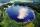Across the circle lakepasses through its center bridge over the lake. At three different locations on the lake shore are three fishermen A, B, C. Which of fishermen see the bridge under the largest angle?
5. Complementary angles 2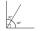Two complementary angles are (x+4) and (2x - 7) find the value of x
6. Plane IIA plane flew 50 km on a bearing 63degrees20 and the flew on a bearing 153degrees20 for 140km. Find the distance between the starting point and the ending point
7. The chordSide of the triangle inscribed in a circle is a chord passing through circle center. What size are the internal angles of a triangle, if one of them is 40°?
8. LadderThe ladder has a length 3.5 meters. He is leaning against the wall so that his bottom end is 2 meters away from the wall. Determine the height of the ladder.
9. Broken treeThe tree is broken at 4 meters above the ground and the top of the tree touches the ground at a distance of 5 from the trunk. Calculate the original height of the tree.
10. HeightIs right that in any right triangle height is less or equal half of the hypotenuse?
11. Type of triangle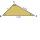How do I find the triangle type if the angle ratio is 2:3:7 ?
12. Chord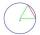Point on the circle is the end point of diameter and end point of chord length of radius. What angle between chord and diameter?
13. DiagonalCan a rhombus have the same length diagonal and side?
14. Center traverseIt is true that the middle traverse bisects the triangle?
15. Obtuse angleWhich obtuse angle is creating clocks at 17:00?
16. Degrees to radiansConvert magnitude of the angle α = 9°39'15" to radians:
17. Isosceles trapezoid v3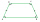In an isosceles trapezoid ABCD is the size of the angle β = 81° Determine size of angles α, γ and δ.